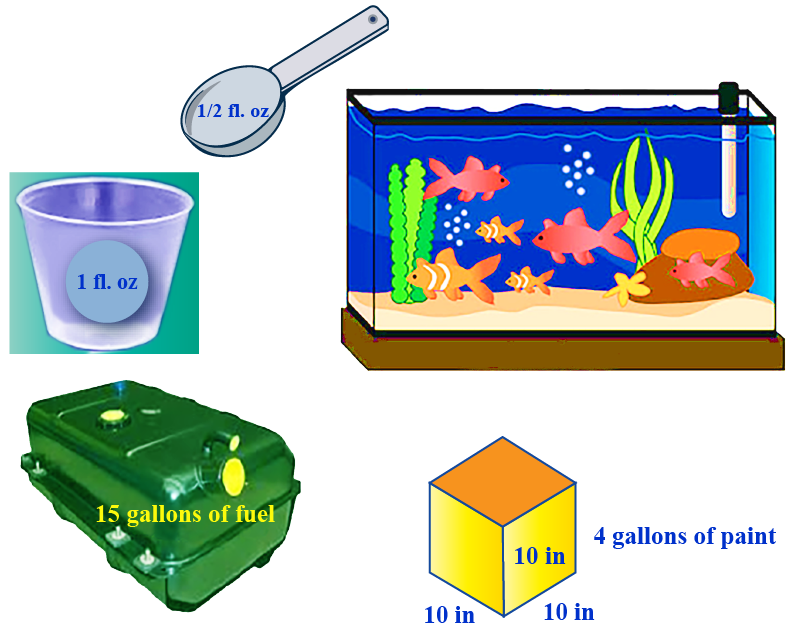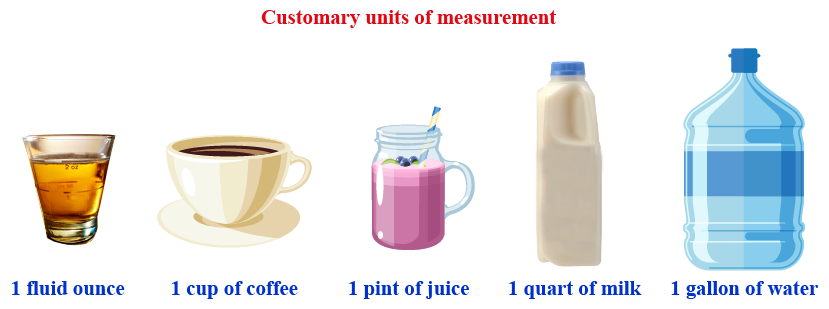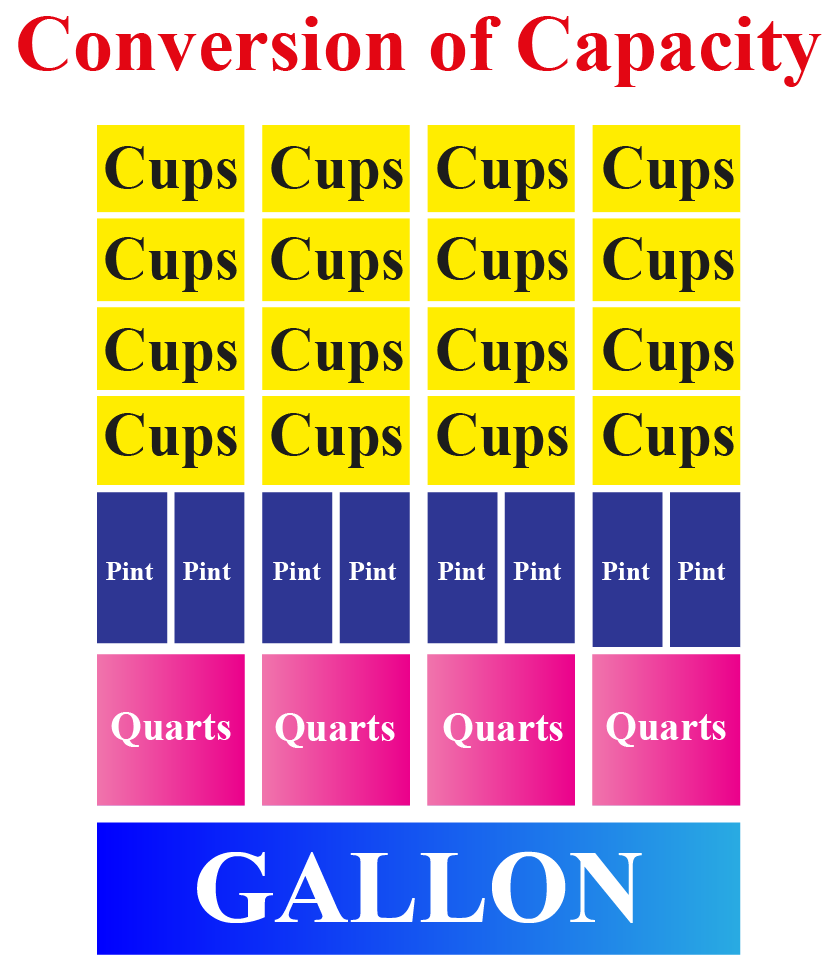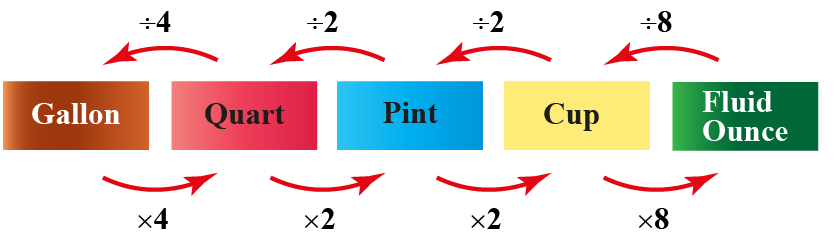# Measuring Capacity

Measuring Capacity

We use spoons, jugs, glasses, beakers, bowls, barrels, bottles, pitchers, cartons, cans, and the like to store liquids.

We take medicine in a fluid ounce.

The capacity of a tablespoon is nearly half a fluid oz.

The capacity of 25 teaspoons is nearly equal to half a cup.

The capacity of a car's fuel tank is about 15 gallons. A fish tank can hold 6 gallons.

A $$10 x 10 x 10$$ inch carton cube can hold 4 gallons of paint.

How do we measure them?

Capacity is the amount of liquid that a container can hold.In this mini-lesson, let us learn about measuring capacity, measure of capacity, the unit of capacity, measure capacity worksheet, and measurement capacity.

## Lesson Plan

 1 What Is Capacity Measurement? 2 Thinking Out of the Box! 3 Important Notes on Measuring Capacity 4 Solved Examples on Measuring Capacity 5 Interactive Questions on Measuring Capacity

## What Is Capacity Measurement?

### Capacity

The term capacity is used for measuring the volume of a liquid.

The amount of liquid that any container can hold is called its capacity.

### Capacity Measurement

The American metric system is also called the United States Customary Units (USCS).

USCS follows 5 customary units to measure the capacity.## How to Measure Capacity?

To get the idea to measure capacity, we need to know the objects that hold liquids around us.

Units of capacity Example
Fluid ounce syrup or tonic
Cup coffee or tea
Pint juice jar
Quart milk carton or water jar
Gallon petrol or gasoline

### Estimation

Let's find what most likely could be used for the capacity measurement by mere estimation.

Capacity Units Estimation
A perfume spray can hold gallons / pints pints
An eyedropper is closer to a cup / a quart cup
A mop bucket has gallons / pints gallons
A tea kettle holds in cups / gallons cups
A water pool measured in quarts / gallons gallons
A mayonnaise jar quarts / pints pints

## Units of Capacity

There are 5 customary units to measure capacity.

A fluid ounce is the smallest unit of measuring capacity and the gallon is the largest unit.

 1 cup = 8 fl. oz

### Gallon Chart

Use this gallon chart to make the conversions of customary units.

###This gallon chart shows us that 1 gallon = 4 quarts  = 8 pints = 16 cups.

###You can multiply or divide according to the known unit and the required unit.

For Example:

1. $$4 \text{ quarts} = \text{____ pints }$$

From the chart we know that

\begin{align}1 \text{ quart} &= 2 \text{ pints}\\4 \text{ quarts} &= 4\times 2 \text{ pints}\\ &= 8 \text{ pints}\end{align}

2. $$6 \text{ pints} = \text{_____ quarts}$$

From the chart, we know that

\begin{align}1 \text{ pint} &= \dfrac{1}{2} \text{ quart}\\\\6 \text{ pints} &= 6\div 2 \\\\&= 3 \text{ quarts}\end{align}

 8 fl oz 1 cup 16 fl oz 1 pint 32 fl oz 1 quart 128 fl oz 1 gallonThink Tank
• What could be the capacity of an Olympic-size swimming pool?
• Ron has 3 cups with him. If he has 4 pints of coffee in a cup, how can he distribute them evenly in 3 cups?

## Measure Capacity

### Measuring Capacity Example

Sophie made 8 quarts of orange juice. She divided it into an equal number of cups. How many cups would she have used?

From the gallon chart, we understand that 4 quarts = 16 cups

Thus 8 quarts of juice will be divided into 32 cups.

$$\therefore$$ she has used 32 cups to pour the orange juice.

### Comparing Capacity Example

A tall glass can hold 4 pints and a big mug can hold 50 fluid oz. Which one holds more?

We know that 4 pints  = 8 cups

and 8 fl. oz = 1 cup.

\begin{align} 50 \text { fl. oz} &= \dfrac{1}{8}\times 50\\&= 6 \dfrac{1}{4} \text{ cups}\end{align}

Thus on comparison we find that

$8 \text{ cups} > 6 \dfrac{1}{4} \text{ cups}$

$$\therefore$$ the tall glass holds more.

### Measurement Capacity

Try solving the simulation below. Adjust the slider to measure the desired amount of gallon.

Click on the measuring beakers and verify how many beakers would you use to fill the gallon jar.

## Solved Examples

 Example 1

The physician prescribed Charlie to take half-fl. oz of medicine twice a day for 4 days. How many cups would he have taken in 4 days?

Solution

In a day he has to take

$$(2\times \dfrac{1}{2}) \text{ fl.oz} = 1 \text{ fluid oz per day}$$

In 4 days he would have taken:

$$4\times 1 = 4 \text{ fluid oz. per day}$$

We know that

\begin{align}8 \text{ fl.oz} &= 1 \text{ cup}\\4 \text{ fl.oz} &= \dfrac{4}{8} \text{cup} \\&= \dfrac{1}{2}\end{align}

 $$\therefore$$Charlie would have taken$$\dfrac{1}{2}$$ a cup of medicine in 4 days.
 Example 2

Tom buys 3 gallons of paint and uses 2 quarts to paint the walls in the living room and 3 quarts to paint his garden fence. How much paint is he left with?

Solution

 1 gallon = 4 quarts

3 gallons = 12 quarts

After painting the wall, the remaining paint:

$$= 12 - 2 = 10$$ quarts.

After painting the fence, the remaining paint:

$$= 10 -3 = 7$$ quarts.

 $$\therefore$$ The left over paint = 7 quarts.
 Example 3

John consumes 20 cups of water in the first 2 days of a week. Going at the same rate, how many quarts would he have consumed at the end of the week?Solution

 1 quart = 4 cups

If 4 cups = 1 quart then

20 cups $$= 20 \div 4 = 5$$ quarts

In 2 days, he consumed 5 quarts.

Then in 7 days he consumed: \begin{align}\\&= \dfrac{5}{2} \times 7\\ &= \dfrac{35}{2} \\&= 17\dfrac{1}{2}\text{ quarts}\end{align}

 $$\therefore$$ John would have consumed $$17\dfrac{1}{2}$$ quarts of water.

## Interactive Questions

Here are a few activities for you to practice. Select/Type your answer and click the "Check Answer" button to see the result.

## Let's Summarize

The mini-lesson targeted the fascinating concept of measuring capacity. The math journey around measuring starts with what a student already knows, and goes on to creatively crafting a fresh concept in the young minds. Done in a way that not only it is relatable and easy to grasp, but also will stay with them forever. Here lies the magic with Cuemath.

At Cuemath, our team of math experts is dedicated to making learning fun for our favorite readers, the students!

Through an interactive and engaging learning-teaching-learning approach, the teachers explore all angles of a topic.

Be it worksheets, online classes, doubt sessions, or any other form of relation, it’s the logical thinking and smart learning approach that we, at Cuemath, believe in.

## 1. What units do we use to measure capacity?

We use the 5 main customary units: fluid ounce, cups, pint, quart, and gallon.

## 2. What does capacity measure?

Capacity measures the amount of liquid occupied by the container it holds.

## 3. What is capacity measured in?

Capacity is measured in 5 customary units. fluid ounce, cups, pint, quart, and gallon.

## 4. Which measurement best describes the capacity of a kitchen sink?

A kitchen sink is reasonably measured in gallons.